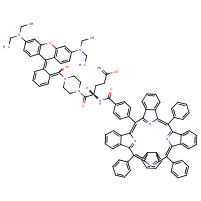Structure InformationCompound Identification

SMILES

CCN(CC)C1=CC2=C(C=C1)C(C1=C(O2)C=C(C=C1)N(CC)CC)=C1C=CC=CC1=C(O)N1CCN(CC1)C(=O)[C@H](CCC(N)=O)N=C(O)C1=CC=C(C=C1)C1=C2N=C(C3=CC=CC=C23)C(=C2N=C(C3=CC=CC=C23)C(=C2N=C(C3=CC=CC=C23)C(=C2N=C1C1=CC=CC=C21)C1=CC=CC=C1)C1=CC=CC=C1)C1=CC=CC=C1

InChIKey

InChIKey=WCZKUIBPWWHFAU-UQKRQPIVSA-N

Formula

C98H82N10O5

Mass

1479.799

### Export to:

Taxonomic Classification

Taxonomy Tree

Kingdom

Organic compounds

Superclass

Organoheterocyclic compounds

Class

Tetrapyrroles and derivatives

Subclass

Porphyrins

Intermediate Tree Nodes

Not available

Direct Parent

Porphyrins

Alternative Parents

Molecular Framework

Aromatic heteropolycyclic compounds

Substituents

Porphyrin - Dibenzopyran - Xanthene - N-acyl-alpha amino acid or derivatives - Alpha-amino acid amide - Diaryl ether - Alpha-amino acid or derivatives - Benzopyran - 1-benzopyran - Isoindole or derivatives - Isoindole - O-quinodimethane - Dialkylarylamine - Tertiary aliphatic/aromatic amine - Monocyclic benzene moiety - Piperazine - 1,4-diazinane - Benzenoid - Fatty amide - Fatty acyl - Heteroaromatic compound - Pyrrole - Tertiary carboxylic acid amide - Amino acid or derivatives - Carboxamide group - Tertiary amine - Primary carboxylic acid amide - Alkanolamine - Carboximidic acid - Carboximidic acid derivative - Carboxylic acid derivative - Oxacycle - Azacycle - Organic 1,3-dipolar compound - Ether - Propargyl-type 1,3-dipolar organic compound - Organooxygen compound - Amine - Organic nitrogen compound - Organic oxygen compound - Organonitrogen compound - Organic oxide - Hydrocarbon derivative - Carbonyl group - Aromatic heteropolycyclic compound

Description

This compound belongs to the class of organic compounds known as porphyrins. These are compounds containing a fundamental skeleton of four pyrrole nuclei united through the alpha-positions by four methine groups to form a macrocyclic structure.

External Descriptors

Not available